Ask question

# State the third congruence required to prove the congruence of triangles using the indicated postulate. 12210203742.jpg a)bar(OM) ~= bar(TS) b)/_M ~= /_S c)bar(ON) ~= bar(TR) d)/_O ~= /_T# State the third congruence required to prove the congruence of triangles using the indicated postulate. 12210203742.jpg a)bar(OM) ~= bar(TS) b)/_M ~= /_S c)bar(ON) ~= bar(TR) d)/_O ~= /_T

Question
Congruenceasked 2021-01-10
State the third congruence required to prove the congruence of triangles using the indicated postulate.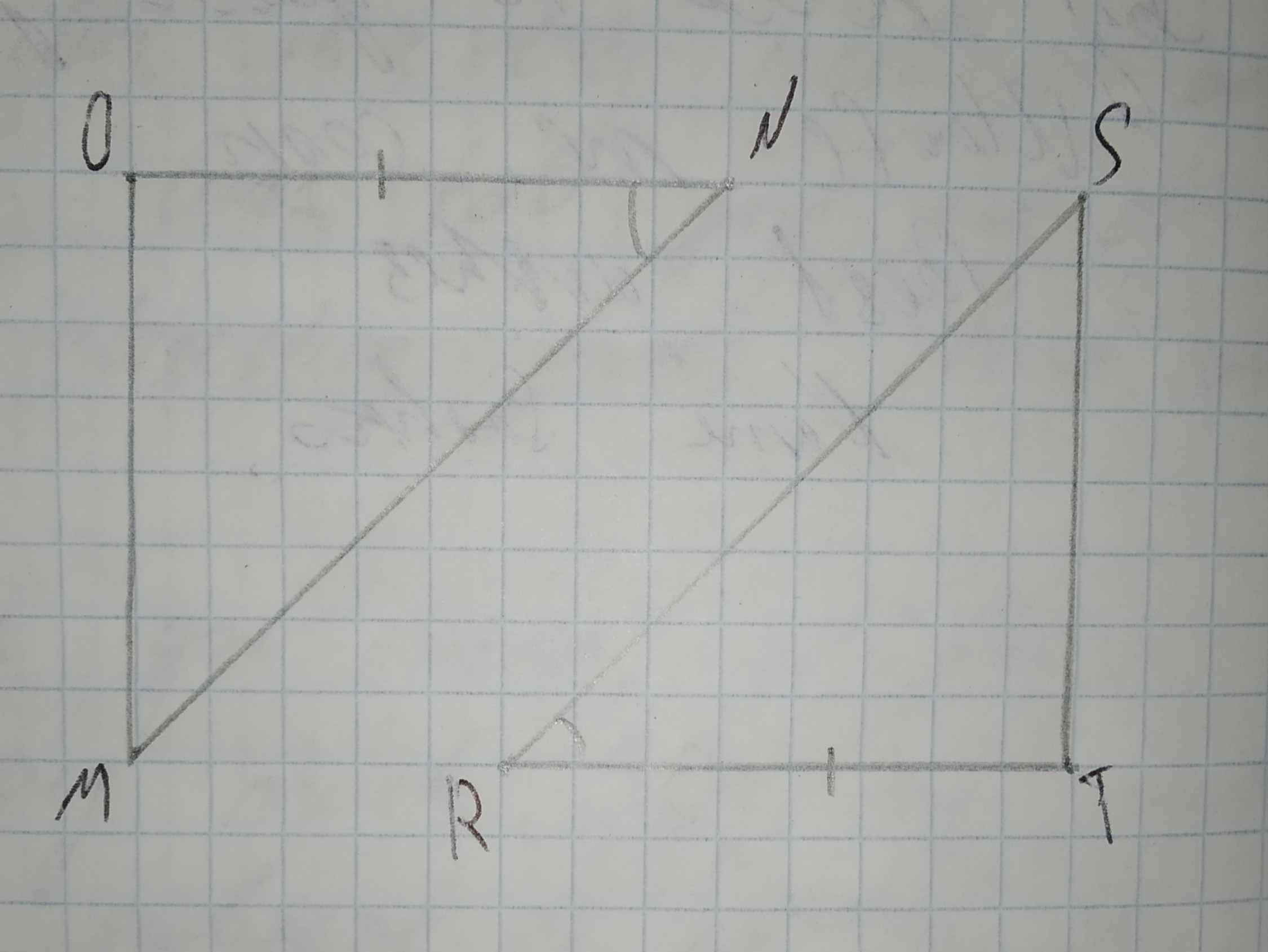a)$$\displaystyle\overline{{{O}{M}}}\stackrel{\sim}{=}\overline{{{T}{S}}}$$
b)$$\displaystyle\angle{M}\stackrel{\sim}{=}\angle{S}$$
c)$$\displaystyle\overline{{{O}{N}}}\stackrel{\sim}{=}\overline{{{T}{R}}}$$
d)$$\displaystyle\angle{O}\stackrel{\sim}{=}\angle{T}$$

## Answers (1)2021-01-11
Given,Step 2
By using ASA postulate, two angles and included side must be equal.
Here,
$$\displaystyle\angle{N}\stackrel{\sim}{=}\angle{R}$$
$$\displaystyle\overline{{{O}{N}}}\stackrel{\sim}{=}\overline{{{R}{T}}}$$
Therefore the third congruence required is,
$$\displaystyle\angle{O}\stackrel{\sim}{=}\angle{T}$$.

### Relevant Questionsasked 2021-02-21
State the third congruence required to prove the congruence of triangles using the indicated postulate.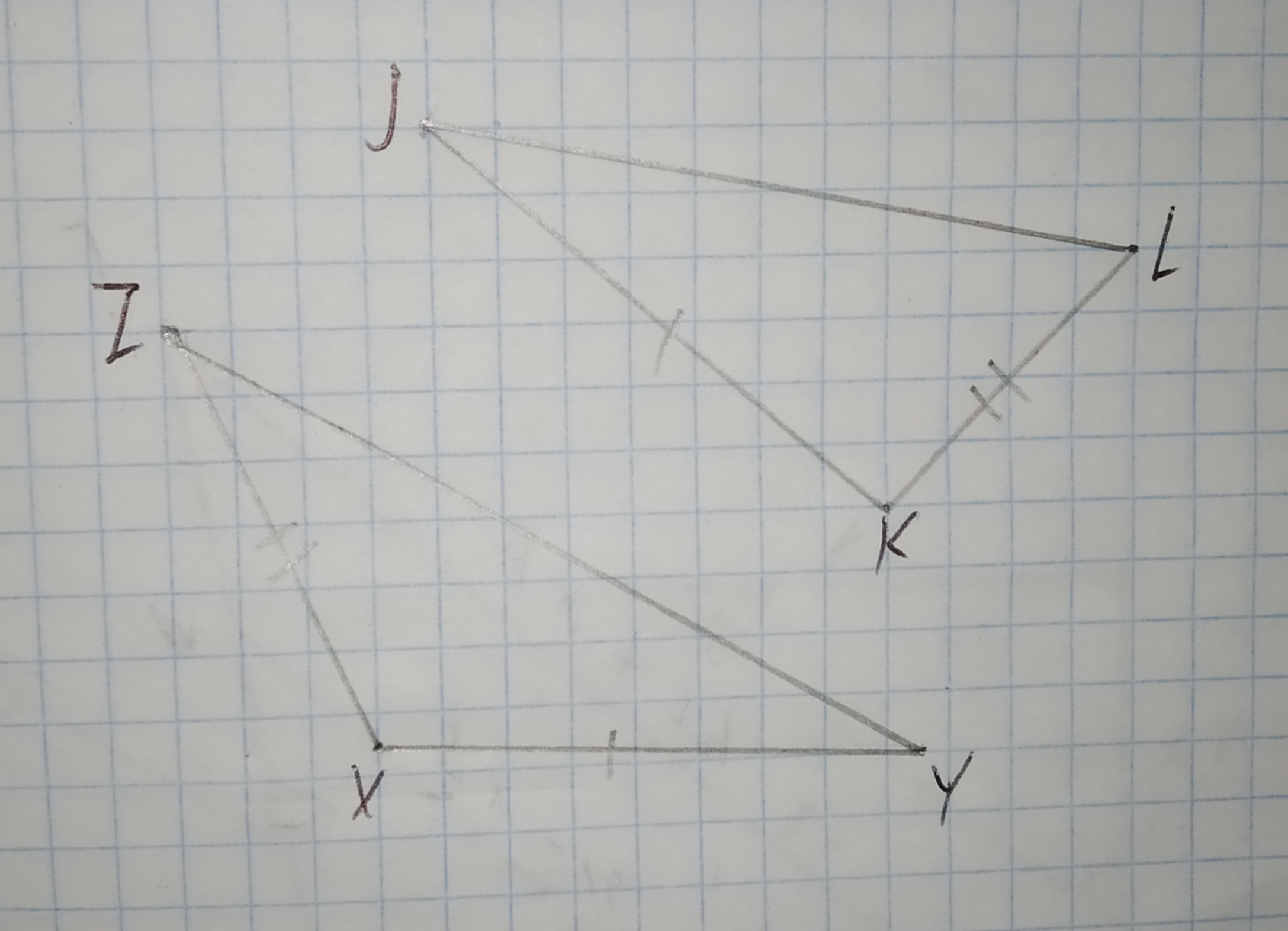a)$$\displaystyle\overline{{{Z}{Y}}}\stackrel{\sim}{=}\overline{{{J}{L}}}$$
b)$$\displaystyle\angle{X}\stackrel{\sim}{=}\angle{K}$$
c)$$\displaystyle\overline{{{K}{L}}}\stackrel{\sim}{=}\overline{{{X}{Z}}}$$
d)$$\displaystyle\angle{Y}\stackrel{\sim}{=}\angle{L}$$asked 2021-03-09

What other information do you need in order to prove the triangles congruent using the SAS Congruence Postulate?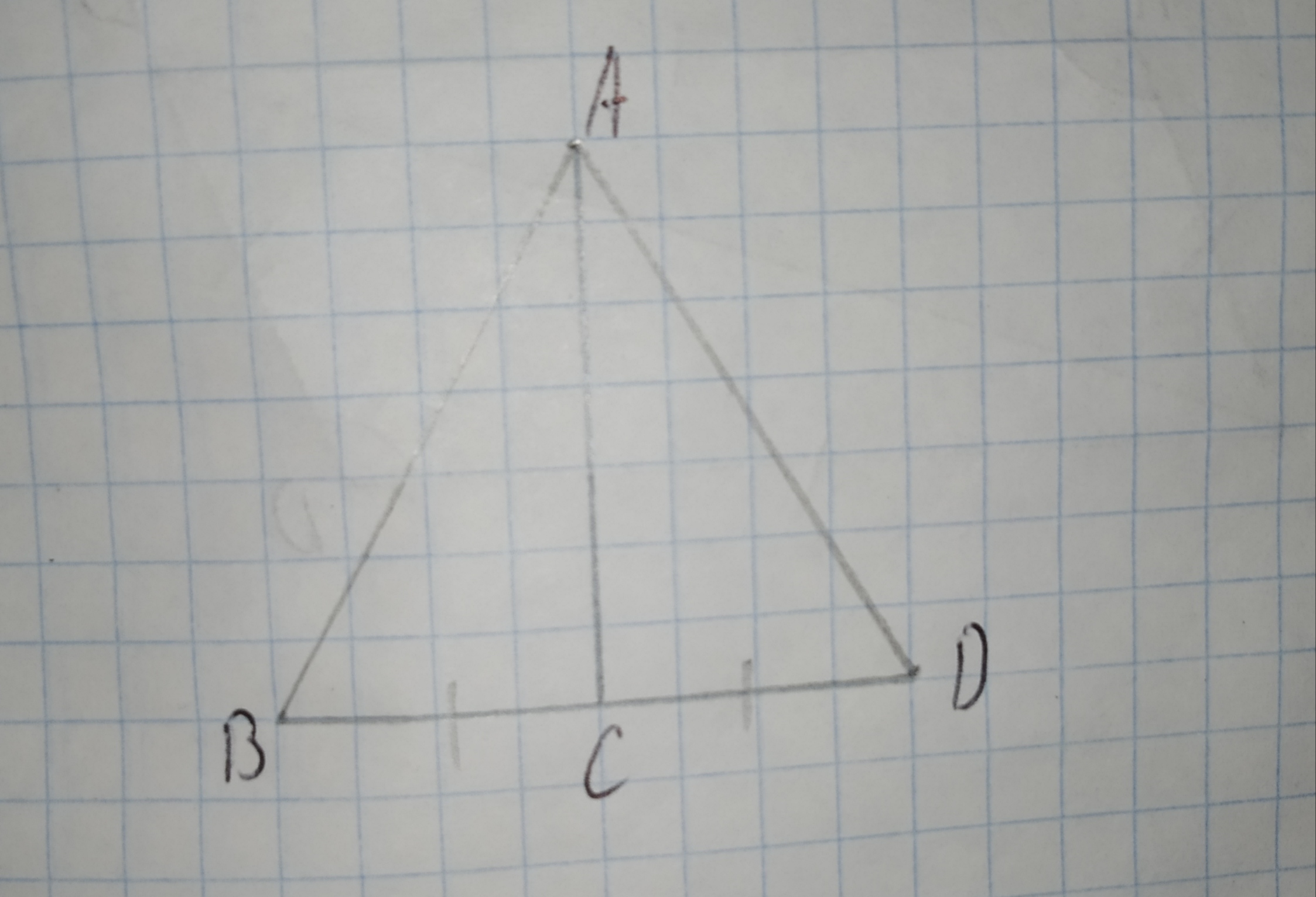A)$$\displaystyle\angle{B}{A}{C}\stackrel{\sim}{=}\angle{D}{A}{C}$$
B)$$\displaystyle\overline{{{A}{C}}}\stackrel{\sim}{=}\overline{{{B}{D}}}$$
C)$$\displaystyle\angle{B}{C}{A}\stackrel{\sim}{=}\angle{D}{C}{A}$$
D)$$\displaystyle\overline{{{A}{C}}}\stackrel{\sim}{=}\overline{{{B}{D}}}$$asked 2020-12-06
With of the following triangle congruence shortcuts could be used to prove PRQ = TRS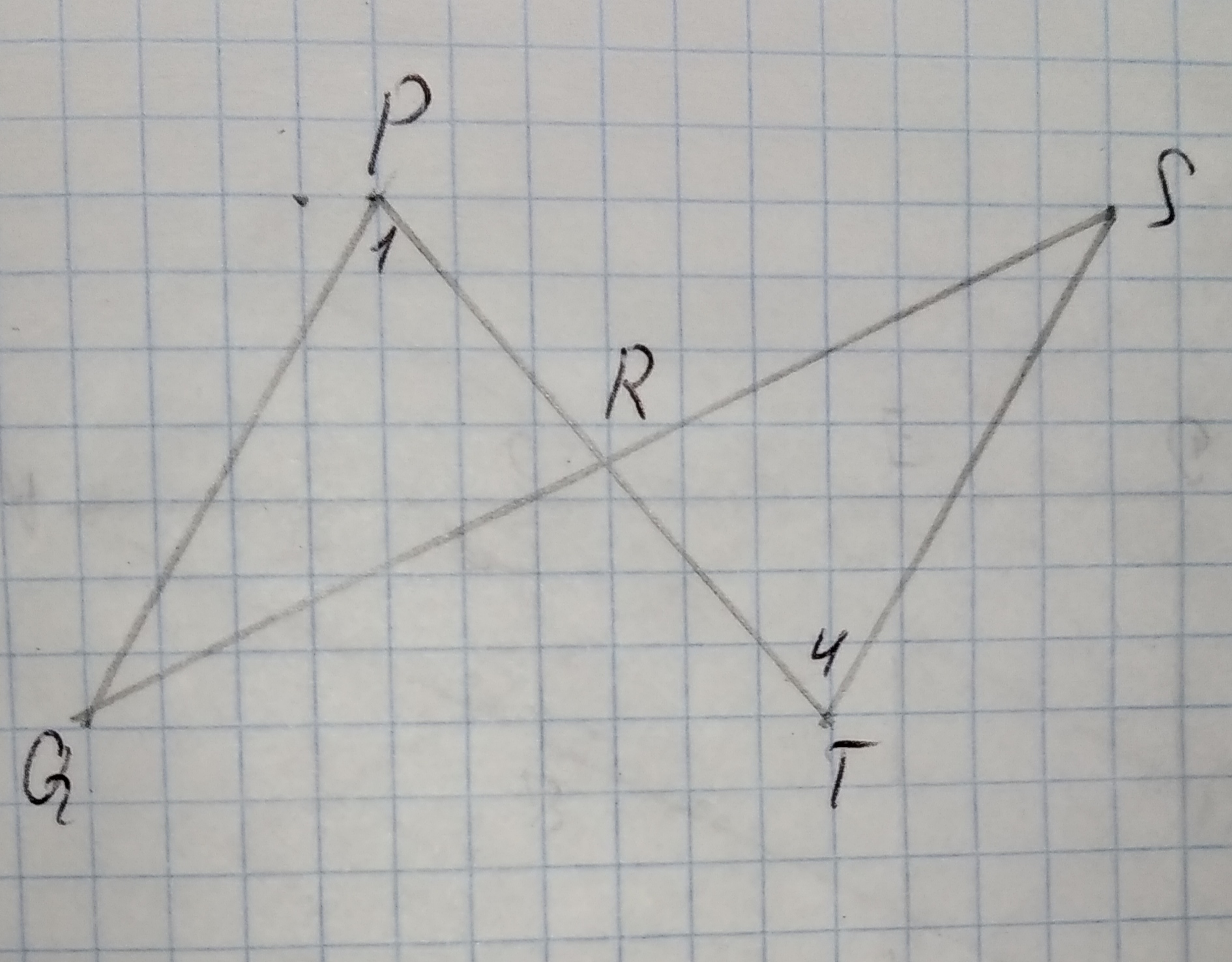Given Data,
$$\displaystyle\angle{Q}\stackrel{\sim}{=}\angle{S}$$
$$\displaystyle\overline{{{Q}{R}}}\stackrel{\sim}{=}\overline{{{S}{R}}}$$
a)Side-Side-Side Postulate (SSS)
b)Side-Angle-Side Postulate (SAS)
c)Angle-Side-Angle Postulate (ASA)
d)Angle-Angle-Side Theorem (AAS)asked 2021-02-27
A Counterexample to the statement, If $$\displaystyle{a}\equiv{b}\pm{o}{d}{m}$$ and $$\displaystyle{c}\equiv{d}\pm{o}{d}{m}$$, where a,b,c,d, and m are integers with c and d positive and $$\displaystyle{m}\geq{2}$$, then $$\displaystyle{a}^{{{c}}}\equiv{b}^{{{d}}}\pm{o}{d}{m}$$.asked 2021-04-02

The postulate or theorem that can be used to prove each pair of triangles congruent.
Given:
The given figure is: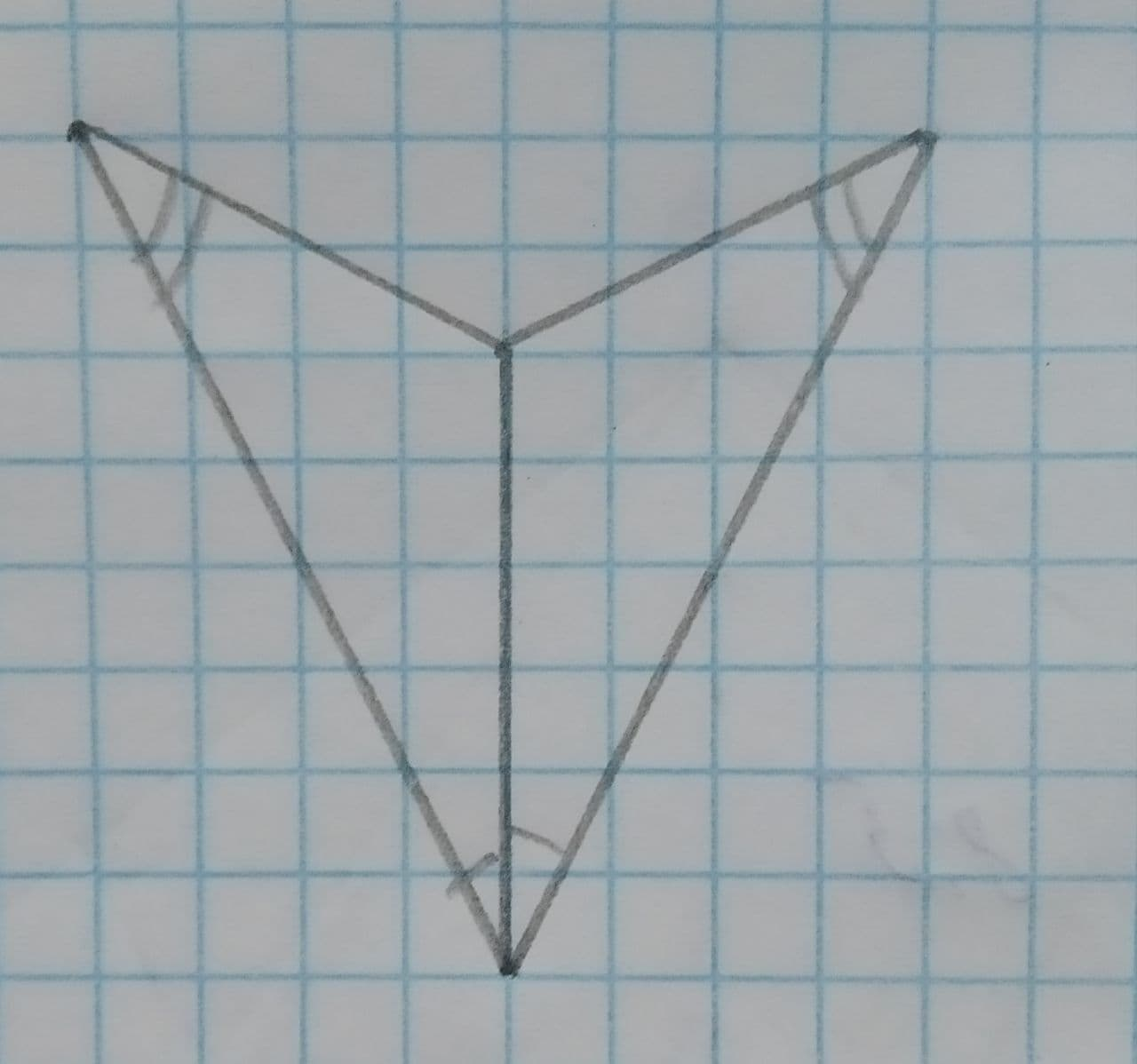asked 2021-02-13
decide whether enough information is given to prove that the triangles are congruent using the SAS Congruence Theorem.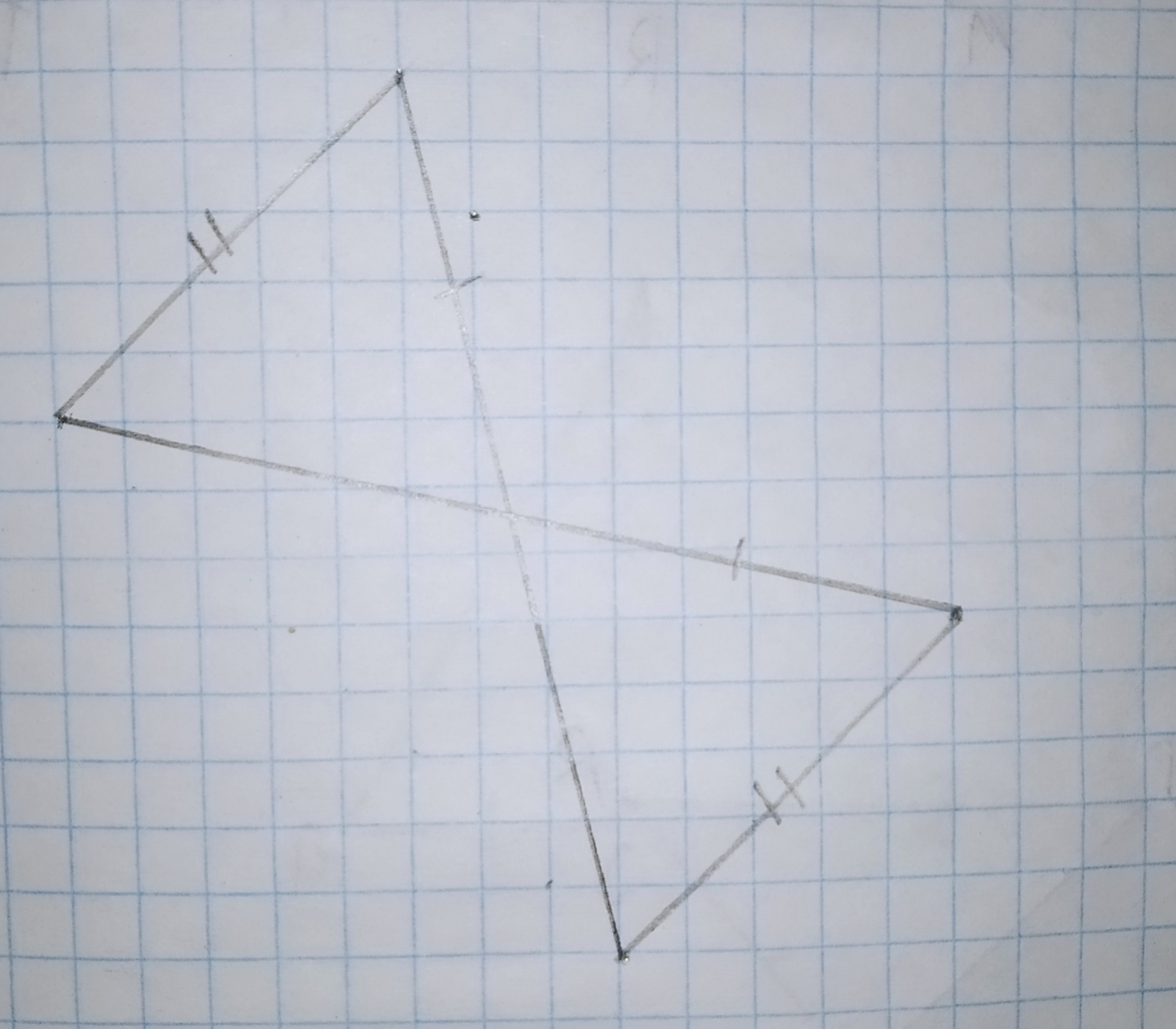asked 2020-12-16
Decide whether enough information is given to prove that the triangles are congruent using the SAS Congruence Theorem.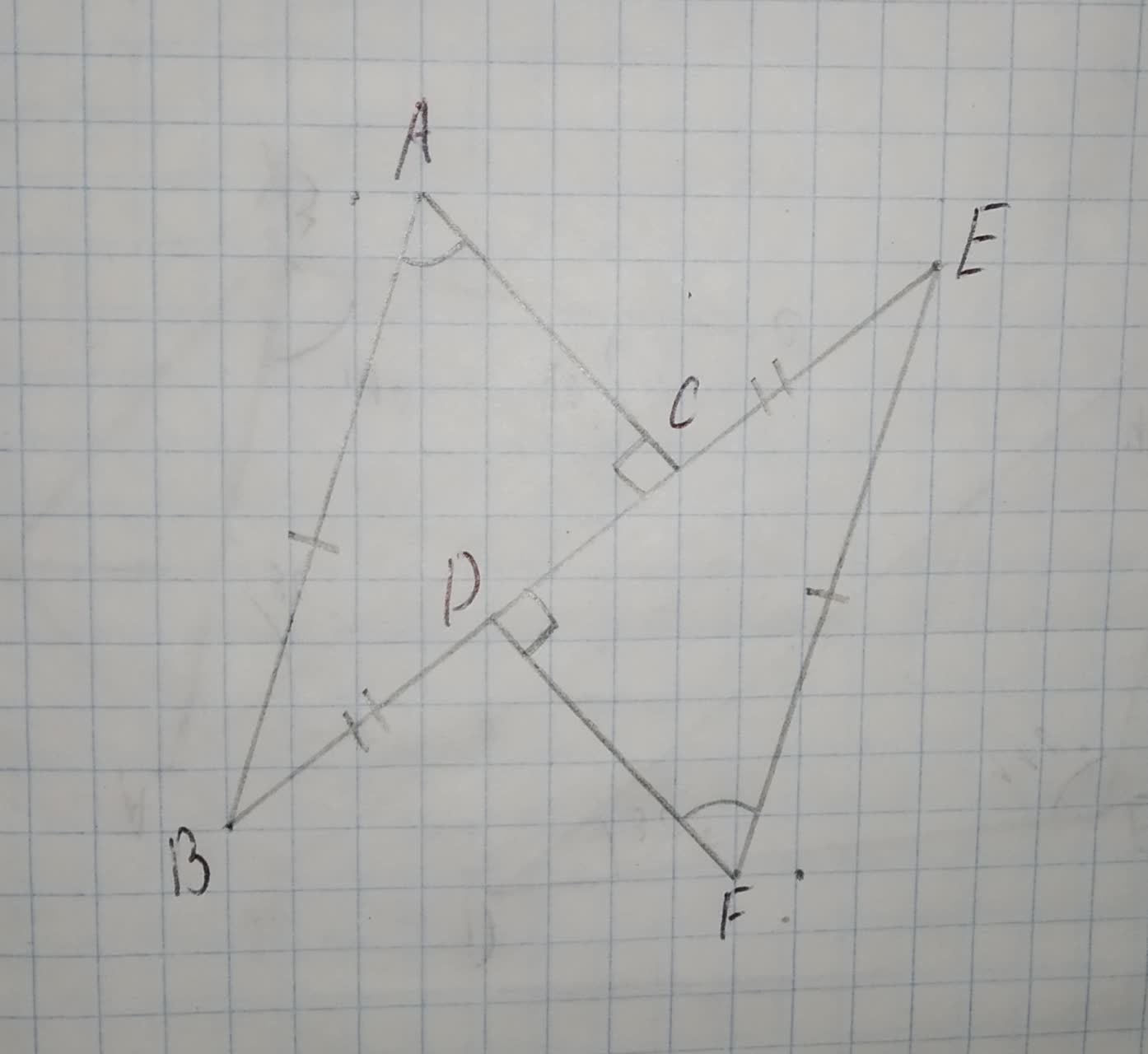asked 2020-11-05
Decide whether the two triangles must be congruent. If so, write the congruence and name the postulate used. If not. write no congruence can be deduced.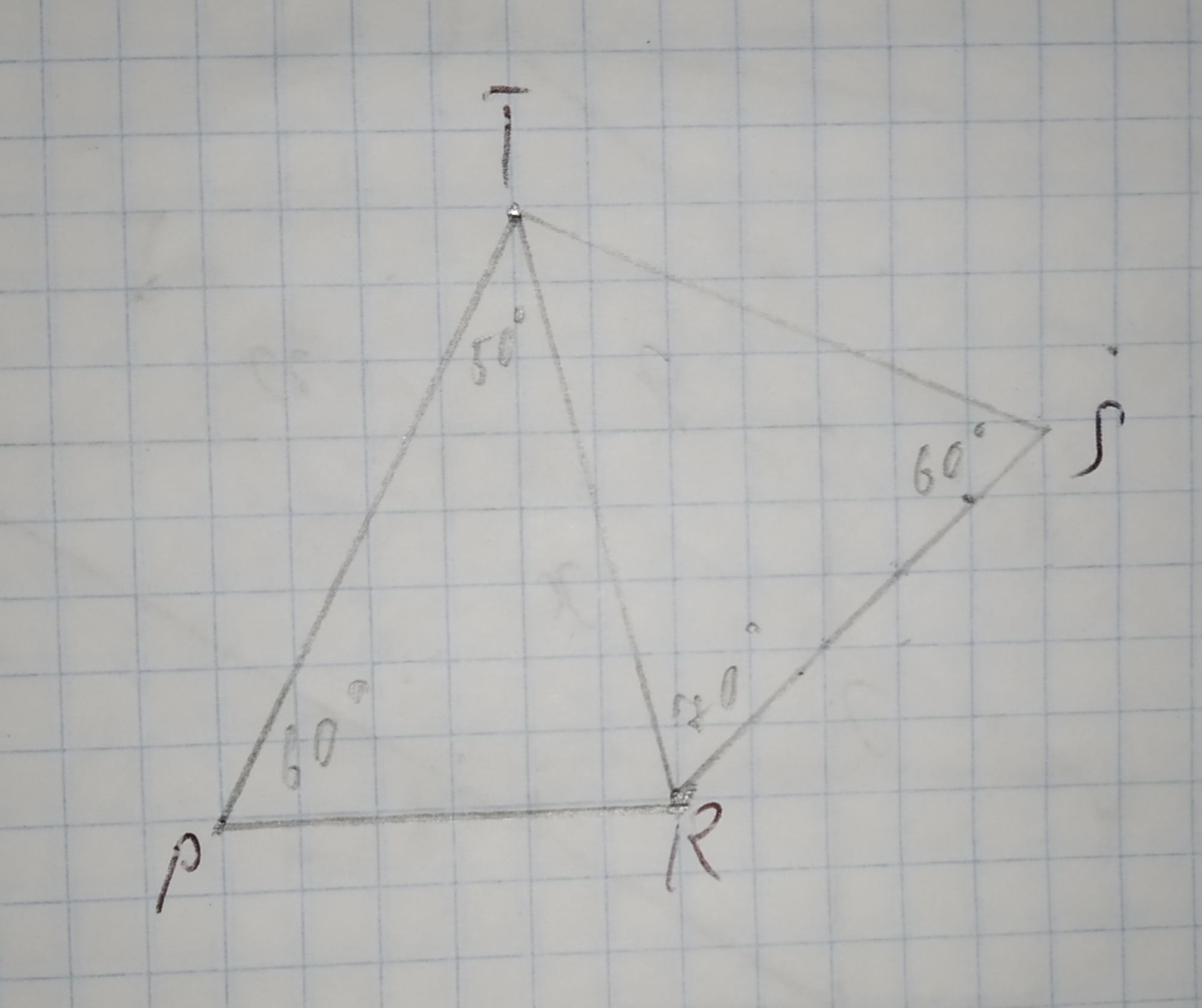asked 2021-04-30
A Counterexample to the statement, If $$\displaystyle{a}{c}\equiv{b}{c}\pm{o}{d}{m}$$, where a,b,c, and m are integers with $$\displaystyle{m}\geq{2}$$, then $$\displaystyle{a}\equiv{b}\pm{o}{d}{m}$$.asked 2020-12-03
Using the diagram below, complete the following congruence statement:
$$\displaystyle\triangle{A}{B}{C}\stackrel{\sim}{=}$$ ?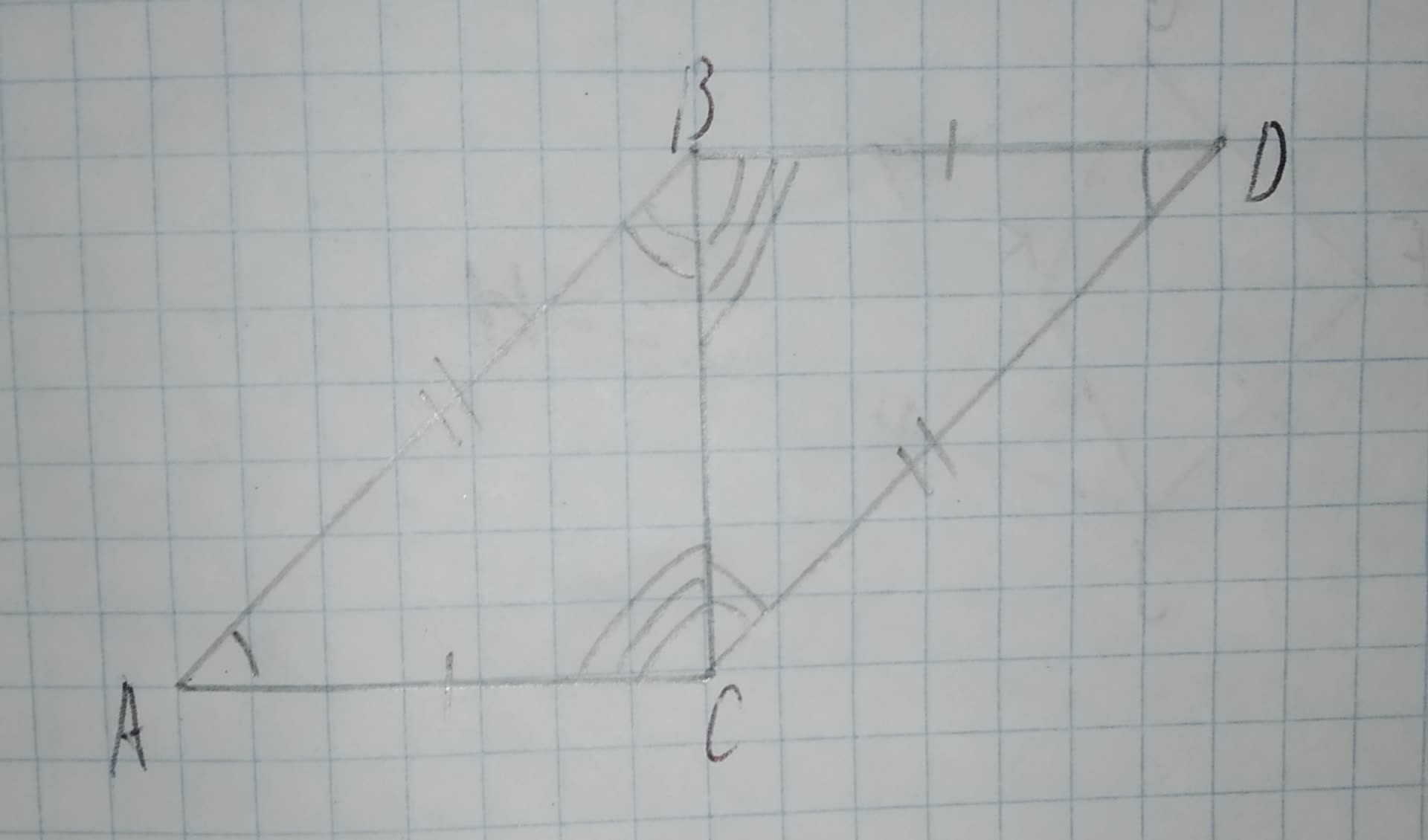a)$$\displaystyle\triangle{D}{B}{C}$$
b)$$\displaystyle\triangle{B}{D}{C}$$
c)$$\displaystyle\triangle{B}{C}{D}$$
d)$$\displaystyle\triangle{D}{C}{B}$$
...